# js 中你不知道的各种循环测速

1. for 循环；

2. while 和 do-while 循环；

3. forEach、map、reduce、filter 循环；

4. for-of 循环；

5. for-in 循环；

```COPYJAVASCRIPT```const len = 100 * 10000;
const arr = [];
for (let i = 0; i < len; i++) {
arr.push(Math.floor(Math.random() * len));
}``````

• 电脑：iMac(10.13.6)；

• 处理器：4.2 GHz Intel Core i7；

• 浏览器：Chrome(89.0.4389.82)## 1. for 循环

for 循环是我们最常用的一种循环方式了，最大的好处就是结构清晰，能够随时 break 停止。

```COPYJAVASCRIPT```console.log('test for');
for (let k = 0; k < 10; k++) {
console.time('for');
let sum = 0;
for (let i = 0; i < len; i++) {
sum += arr[i] % 100;
}
console.timeEnd('for');
}``````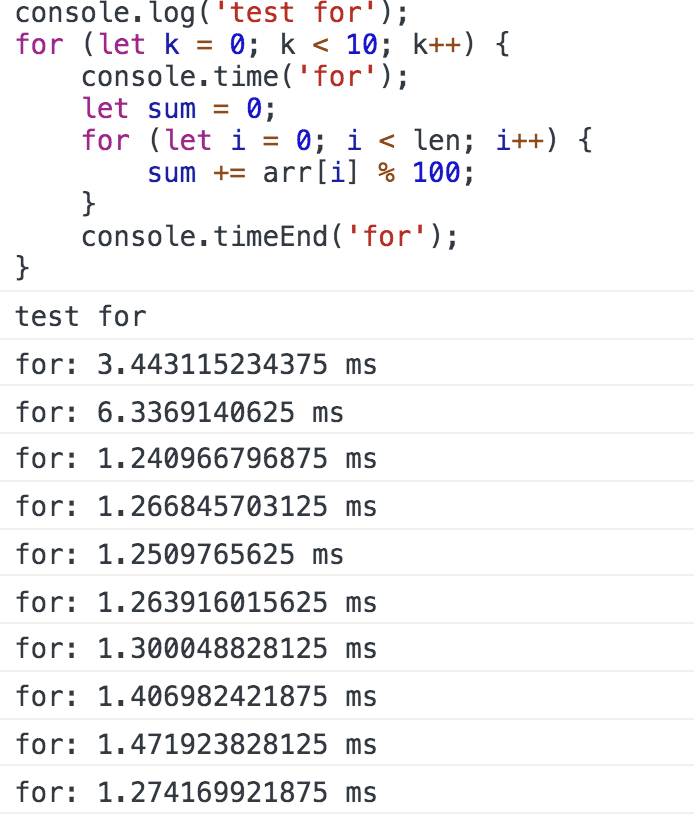## 2. while 循环和 do-while 循环

```COPYJAVASCRIPT```console.log('\ntest while');
for (let k = 0; k < 10; k++) {
console.time('while');
let sum = 0;
let i = 0;
while (i < len) {
sum += arr[i] % 100;
i++;
}
console.timeEnd('while');
}
console.log('\ntest do-while');
for (let k = 0; k < 10; k++) {
console.time('do-while');
let sum = 0;
let i = 0;
do {
sum += arr[i] % 100;
i++;
} while (i < len);
console.timeEnd('do-while');
}``````

while 循环和 do-while 循环的结果几乎一样，我们只看下 while 循环在浏览器上运行的结果：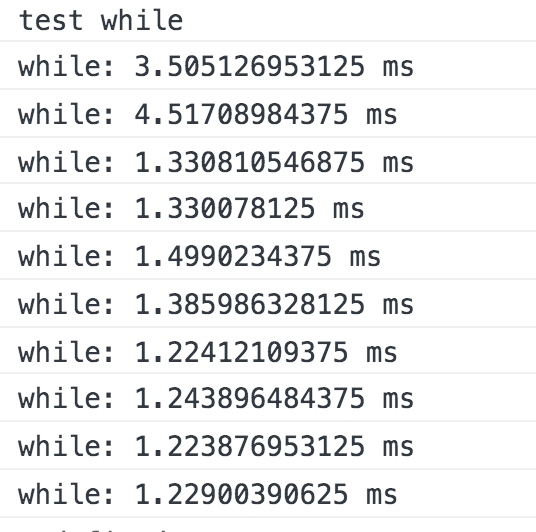## 3. forEach、map 和 reduce 循环

### 3.1 forEach 的简要介绍

```COPYJAVASCRIPT```[1, 2, 3, 4, 5].forEach((item) => {
console.log(`before return: \${item}`);
if (item % 3 === 0) {
return;
}
console.log(`after return: \${item}`);
});``````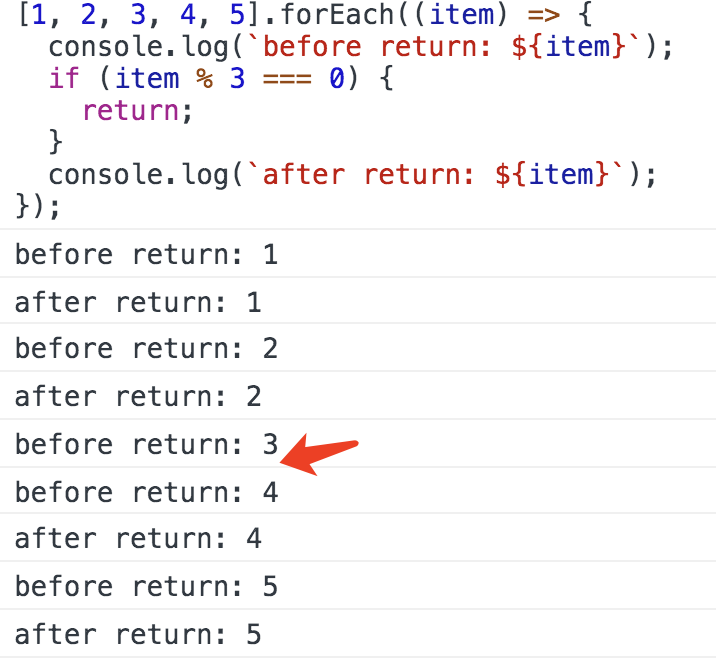```COPYJAVASCRIPT```try {
[1, 2, 3, 4, 5].forEach((item) => {
console.log(`before return: \${item}`);
if (item % 3 === 0) {
throw new Error('break forEach');
}
console.log(`after return: \${item}`);
});
} catch (e) {}``````### 3.3 forEach 等的测速

```COPYJAVASCRIPT```// forEach 的测试：
console.log('\ntest forEach');
for (let k = 0; k < 10; k++) {
console.time('forEach');
let sum = 0;
arr.forEach((item) => {
sum += item % 100;
});
console.timeEnd('forEach');
}``````
```COPYJAVASCRIPT```// map 的测试：
console.log('\ntest map');
for (let k = 0; k < 10; k++) {
console.time('map');
let sum = 0;
arr.map((item) => {
sum += item % 100;
});
console.timeEnd('map');
}``````
```COPYJAVASCRIPT```// reduce 的测试：
console.log('\ntest reduce');
for (let k = 0; k < 10; k++) {
console.time('reduce');
let sum = 0;
arr.reduce((_, item) => {
sum += item % 100;
}, 0);
console.timeEnd('reduce');
}``````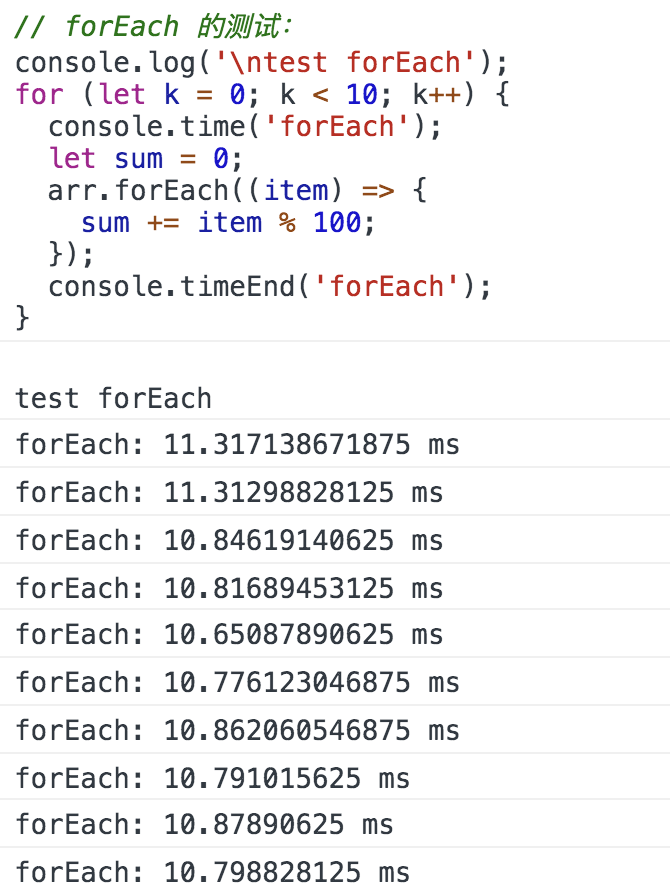## 4. for-of

ES6 借鉴 C++、Java、C# 和 Python 语言，引入了 for...of 循环，作为遍历所有数据结构的统一的方法。

### 4.1 for-of 的简要介绍

for...of 循环可以使用的范围包括数组、Set 和 Map 结构、某些类似数组的对象（比如 arguments 对象、DOM NodeList 对象）、后文的 Generator 对象，以及字符串。

for-of 拿到的就是 value 本身，而 for-in 则拿到的是 key，然后通过 key 再获取到当前数据。

```COPYJAVASCRIPT```const fruits = ['apple', 'banana', 'orange', 'lemon'];

for (const value of fruits) {
console.log(value); // 'apple', 'banana', 'orange', 'lemon'
}``````### 4.2 for-of 的循环测速

```COPYJAVASCRIPT```console.log('\ntest for-of');
for (let k = 0; k < 10; k++) {
console.time('for-of');
let sum = 0;
for (const value of arr) {
sum += value % 100;
}
console.timeEnd('for-of');
}``````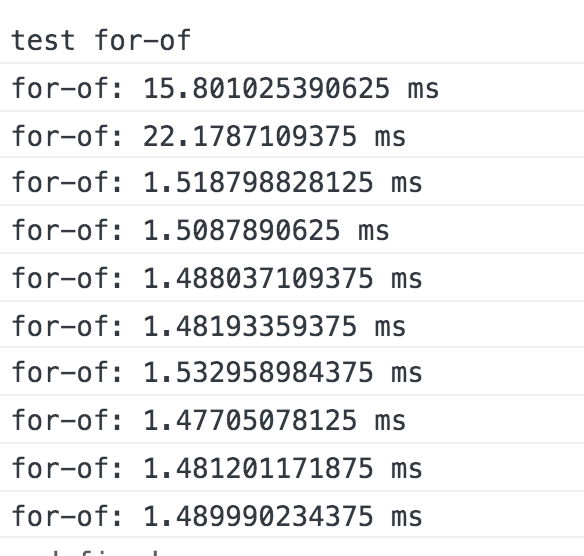## 5. for-in 循环

for-in 通常用于 object 类型的循环，但也可以用来循环数组，毕竟所有数据类型的祖先都是 object 类型。

```COPYJAVASCRIPT```console.log('\ntest for-in');
for (let k = 0; k < 10; k++) {
console.time('for-in');
let sum = 0;
for (let key in arr) {
sum += arr[key] % 100;
}
console.timeEnd('for-in');
}``````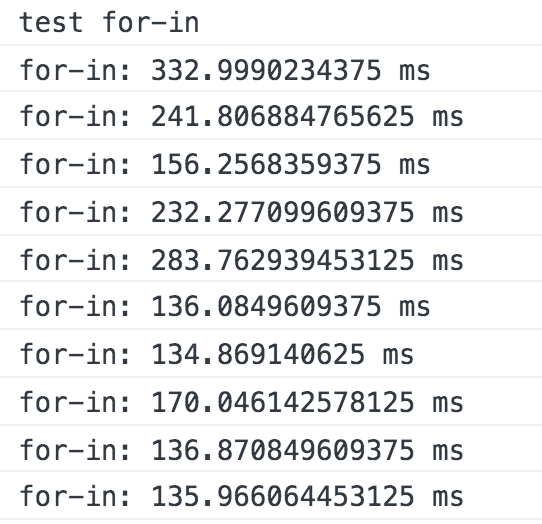for-in 循环的测速数据很惊人，简直是独一档的存在了，最好的时候也至少需要 136ms 的时间。可见 for-in 的循环效率真的很低。```COPYJAVASCRIPT```const obj = {};
for (let i = 0; i < len; i++) {
obj[i] = Math.floor(Math.random() * len);
}
for (let k = 0; k < 10; k++) {
console.time('forEach-values');
let sum = 0;
Object.values(obj).forEach((item) => {
sum += item % 100;
});
console.timeEnd('forEach-values');
}``````

## 6. 总结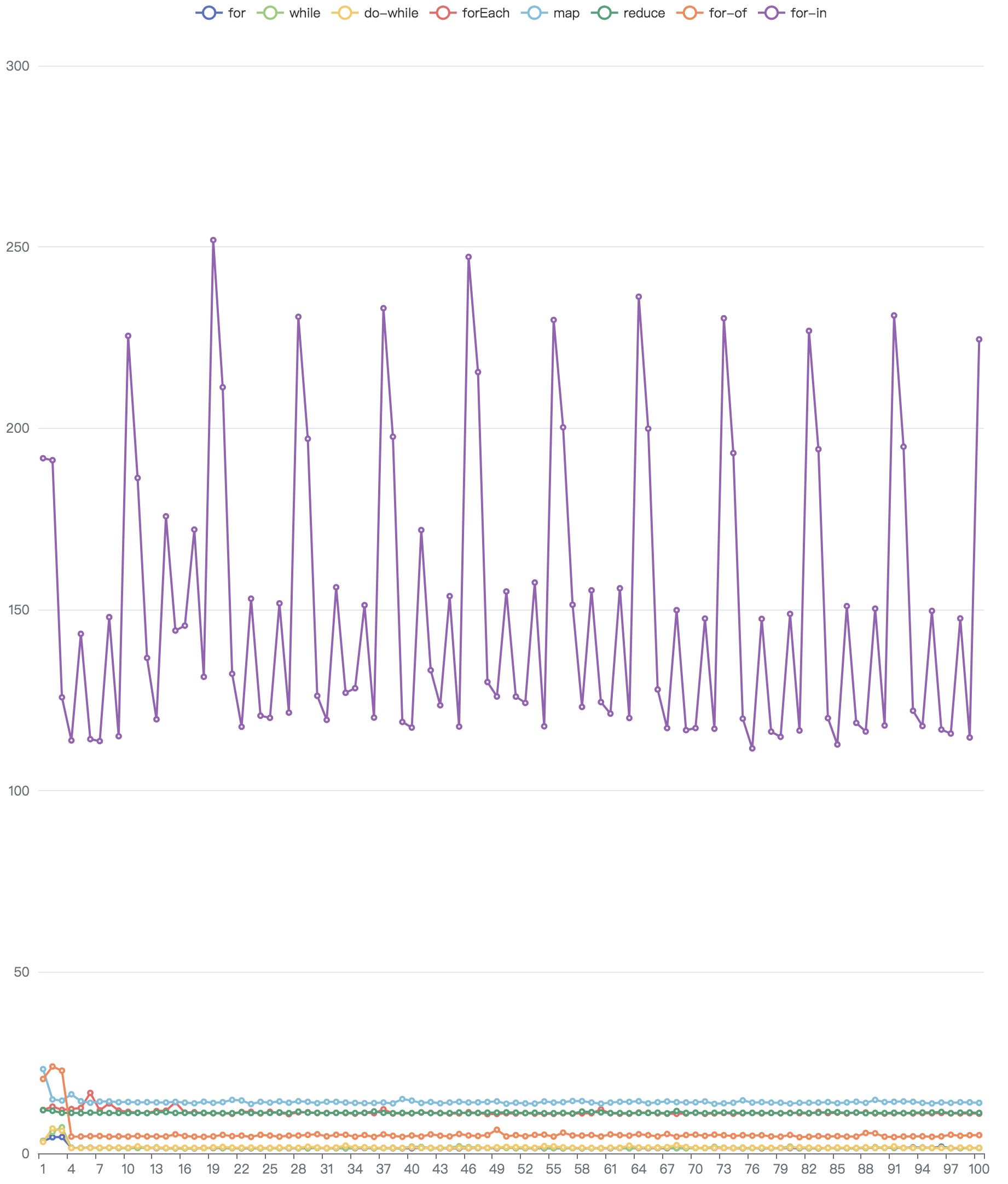• for 循环、while 循环和 d-while 循环的时间最少；

• for-of 循环的时间稍长；

• forEach 循环、map 循环和 reduce 循环 3 者的数据差不多，但比 for-of 循环的时长更长一点；

• for-in 循环所需要的时间最多；### 公众号：### 公众号：